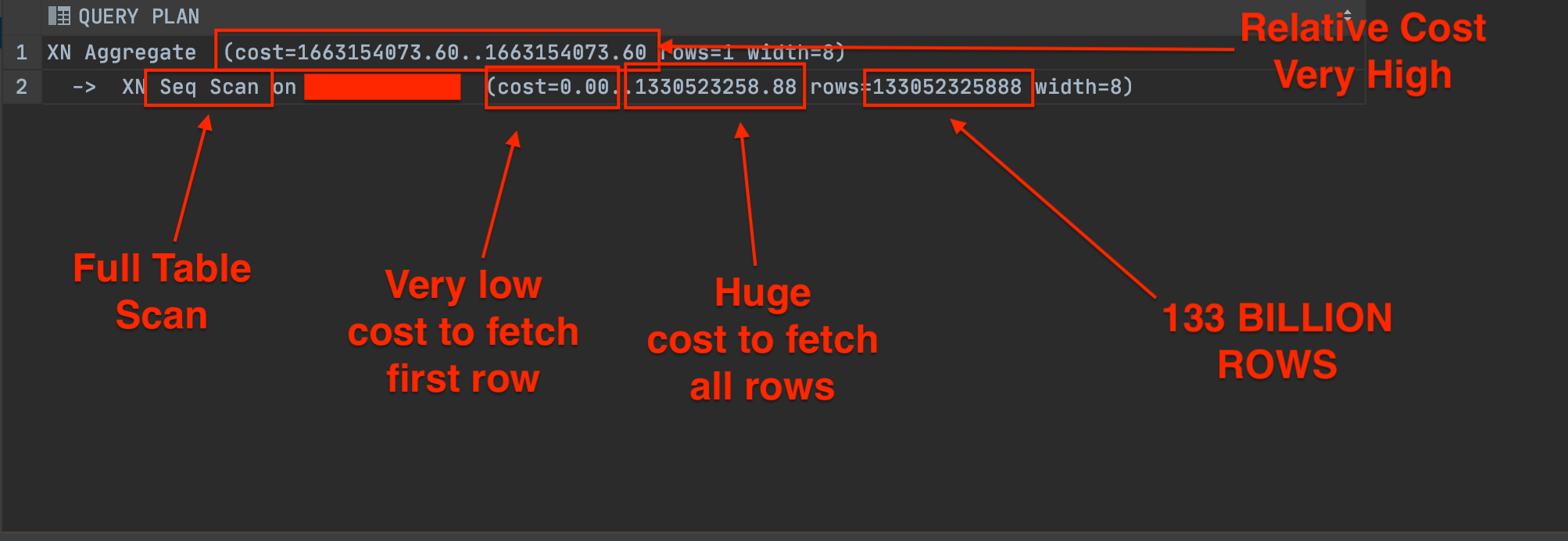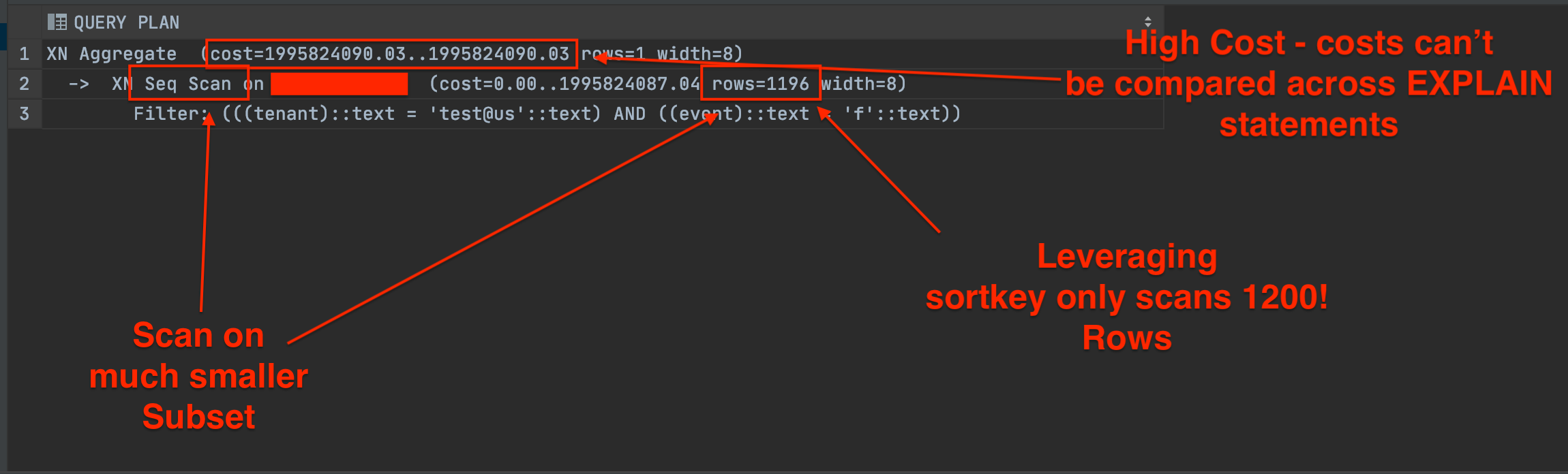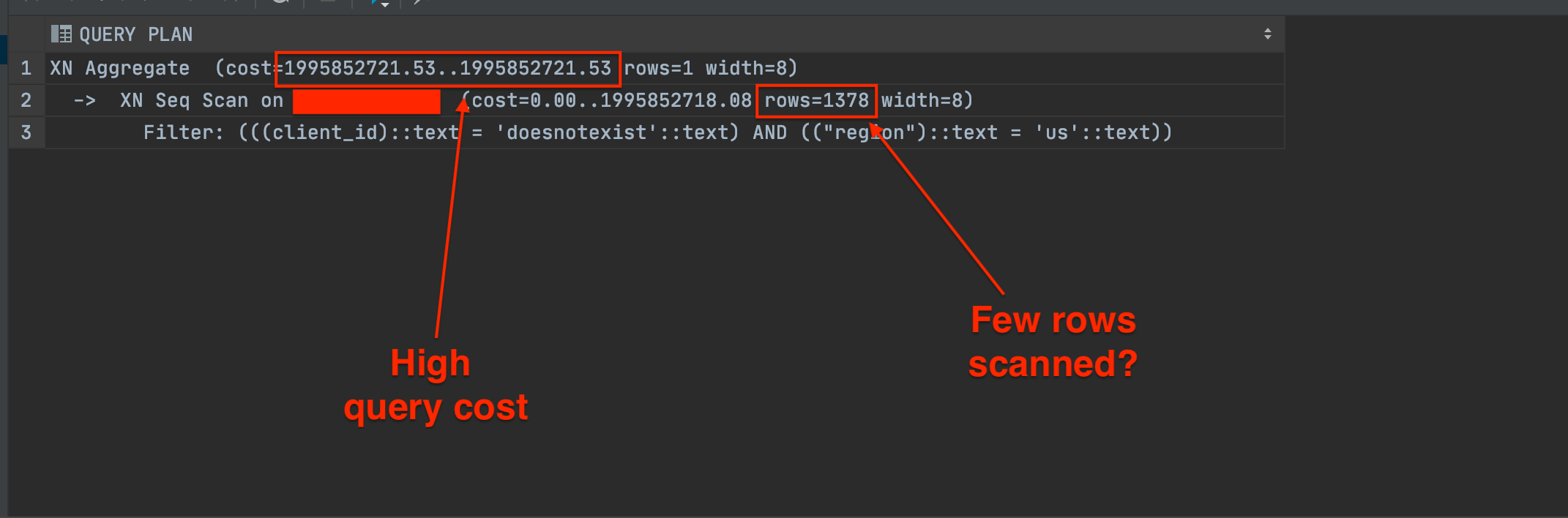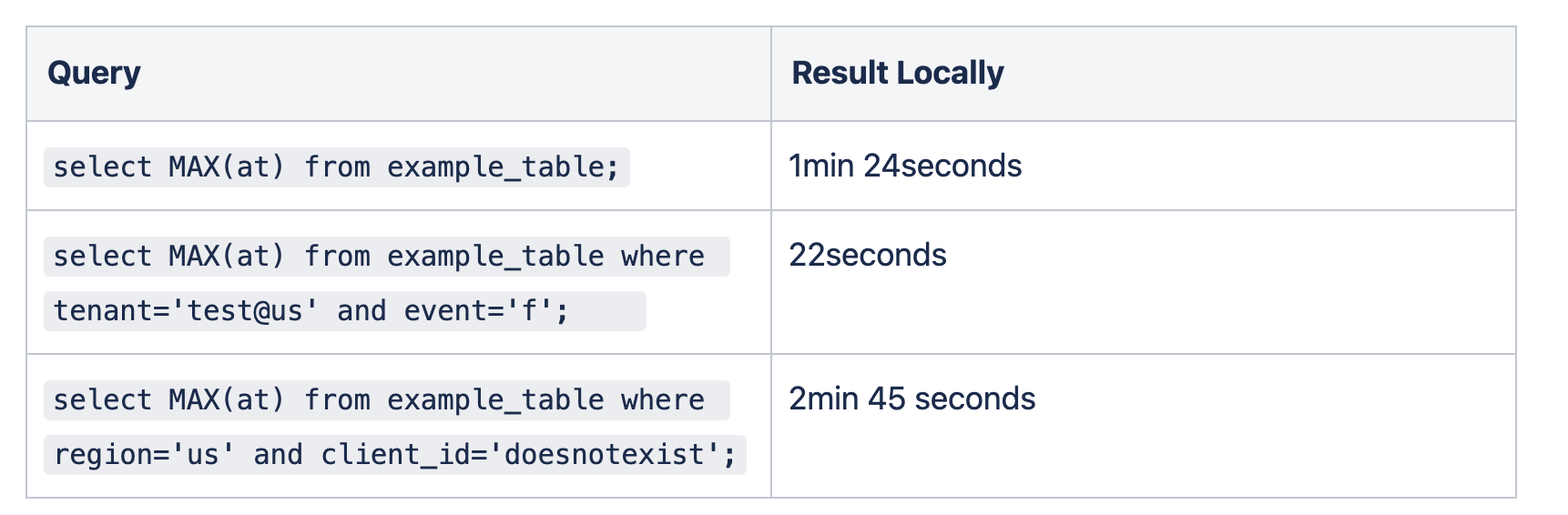Published on

# Redshift Understanding the "EXPLAIN" Statement

Authors
•Name
Danny Mican

You’re developing a query using Amazon Redshift, how do you know if it’s fast or optimized? This tutorial walks through using the EXPLAIN statement to understand query performance using an example query.

# Methodology

The following steps are helpful when using EXPLAIN.

• Understand the table schema.
• Determine sortkey, if any.
• Understand the query through the EXPLAIN statement.

# Determine the table schema

If you have admin access the full schema can be accessed using:

``````SELECT
ddl
FROM
WHERE
schemaname = 'public';
``````

Searching the query above shows the schema for an example table:

``````"CREATE TABLE IF NOT EXISTS ""public"".""example_table"""
(
"	""id"" CHAR(64)   ENCODE zstd"
"	,""tenant"" VARCHAR(512)   ENCODE zstd"
"	,""at"" TIMESTAMP WITHOUT TIME ZONE   ENCODE zstd"
"	,""event"" VARCHAR(64)   ENCODE bytedict"
)
DISTSTYLE KEY
"DISTKEY (""id"")"
SORTKEY (
"	""tenant"""
"	, ""event"""
"	, ""at"""
)
;
``````

# Determine the Table Sortkey

The CREATE table statement above shows the following sortkey:

(`tenant`, `event`, `at`)

Order is important with sortkeys. In the example above, `event` is sorted WITHIN tenants and `at` is sorted within (`tenant`, `event`). This means that a query filtering on `event` will not utilize the sort key speedup since `event` is only filtered within each `tenant`!

Another way to get this information is to query pg_table_def directly:

``````SELECT * FROM pg_table_def WHERE tablename = 'example_table';
``````

The results show the same sortkey (tenant, event, at):# Explain the Query

Now that we have a base understanding of the table structure and sortkey let’s try a query!

Let’s pull the date from the most recent row in example_table:

``````select MAX(at) from example_table;
``````

We run this query but it takes forever…. EXPLAIN can be used to help you understand how redshift is executing the query, the plan for executing the query, and the relative costs of those steps.

The following image shows the results using the raw plan in datagrip:This query is brutal, We are scanning 133 BILLION records to fetch a single row!

# Leveraging Sortkey

Next shows an example of using the sortkey:

``````select MAX(at) from example_table where tenant='test@us' and event='f';
``````

It selects the max event for a specific tenant and event type. The explain statement for this query looks much different:The cost for this is still very high, but redshift has to query much fewer rows to compute the result.

# Predicate Query Missing Sortkey

The final example will show a predicate query that doesn’t leverage the sortkey:What’s interesting here is that the rows do NOT indicate the amount of work being done. Both this query and the previous query have small row values of 1378 and 1196, respectively. But the previous query completes~7.5 times faster!

This stackoverflow post suggests that redshift does not reflect the sortkey in the EXPLAIN statement, and another table needs to be queried to get that information.

# ResultsThe wall clock time indicates leveraging sort keys provide the fastest query times, and the EXPLAIN statement describes why and how that's the case by providing insights into the strategy redshift is using to perform the query! EXPLAIN statements are so lightweight they should be incorporated any time a query is created!